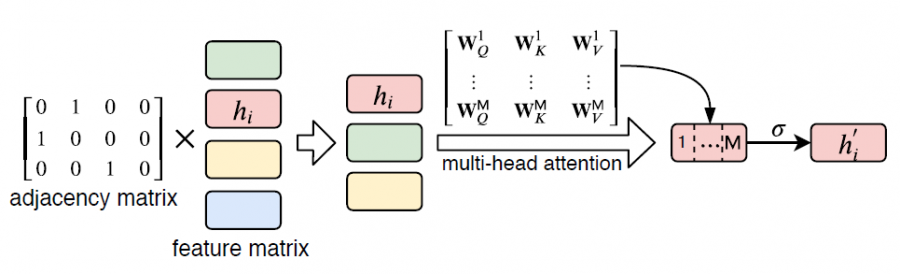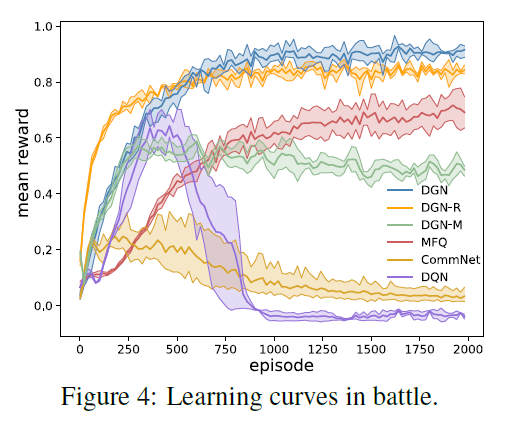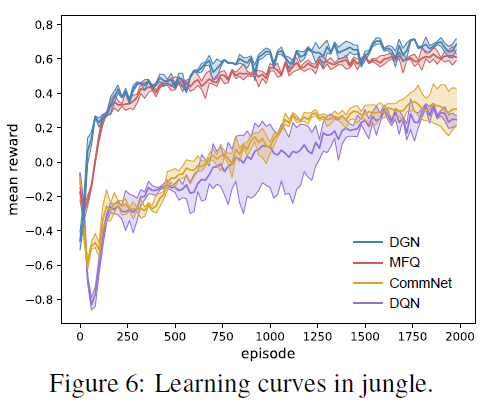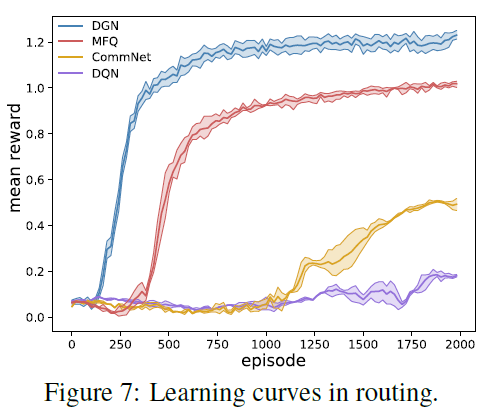# 多智能体强化学习算法

## Graph Convolutional Reinforcement Learning

Posted by MY on August 25, 2020

## 二、解法

### 2.1 graph convolution$\mathcal{L}(\theta)=\frac{1}{\mathrm{S}} \sum_{\mathrm{S}} \frac{1}{\mathrm{N}} \sum_{i=1}^{\mathrm{N}}\left(y_{i}-Q\left(O_{i, \mathcal{C}}, a_{i} ; \theta\right)\right)^{2}$

### 2.2 relation kernel

$\alpha_{i j}^{m}=\frac{\exp \left(\tau \cdot \mathbf{W}_{Q}^{m} h_{i} \cdot\left(\mathbf{W}_{K}^{m} h_{j}\right)^{\top}\right)}{\sum_{k \in \mathbb{B}_{+i}} \exp \left(\tau \cdot \mathbf{W}_{Q}^{m} h_{i} \cdot\left(\mathbf{W}_{K}^{m} h_{k}\right)^{\top}\right)}$

$h_{i}^{\prime}=\sigma\left(\text { concatenate }\left[\sum_{j \in \mathbb{B}_{+i}} \alpha_{i j}^{m} \mathbf{W}_{V}^{m} h_{j}, \forall m \in \mathbb{M}\right]\right)$### 2.3 Temporal Relation Regularization

$\mathcal{L}(\theta)=\frac{1}{\mathrm{S}} \sum_{\mathrm{S}} \frac{1}{\mathrm{N}} \sum_{i=1}^{N}\left(\left(y_{i}-Q\left(O_{i, \mathcal{C}}, a_{i} ; \theta\right)\right)^{2}+\lambda \frac{1}{\mathrm{M}} \sum_{m=1}^{\mathrm{M}} D_{\mathrm{KL}}\left(\mathcal{G}_{m}^{\kappa}\left(O_{i, \mathcal{C}} ; \theta\right) \| \mathcal{G}_{m}^{\kappa}\left(O_{i, \mathcal{C}}^{\prime} ; \theta\right)\right)\right.$

## 三、实验内容## 五、优点

##### Share